Courses

# Class Equations Mathematics Notes | EduRev

## Algebra for IIT JAM Mathematics

Created by: Veda Institute

## Mathematics : Class Equations Mathematics Notes | EduRev

The document Class Equations Mathematics Notes | EduRev is a part of the Mathematics Course Algebra for IIT JAM Mathematics.
All you need of Mathematics at this link: Mathematics

If X is a G-set, X is partitioned by the G-orbits. So if X is ﬁnite,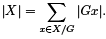(“x ∈ X/G” means you should take one representative x from each orbit, and sum over the set of representatives. This is diﬀerent from the notation “K ∈ X/G” which occurred in the proof of Burnside’s Theorem; there I was actually summing over the set of orbits.) x is a ﬁxed point if gx = x for all g ∈ G. In this case, x is an orbit all by itself. Recall that X G denotes the set of ﬁxed points of X . I can pull these one-point orbits out of the sum above to obtain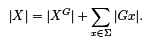In this case, Σ will denote the set of orbits which have more than one point. (I’ll call them nontrivial orbits for short.) Thus, “x ∈ Σ” means that you should take one representative x from each nontrivial orbit.

Example. Here is a set X of 9 points arranged in a square: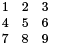Let the group D4 of symmetries of the square act on X . There is one ﬁxed point: 5. There are two nontrivial orbits: {1, 3, 7, 9} and {2, 4, 6, 8}. The equation above says

9 = 1 + (4 + 4).

Lemma. If G is a group, G acts on itself by conjugation:

g ⋆ x = gxg−1 for g, x ∈ G.

Proof. 1 ⋆ x = 1 · x · 1−1 = x for all x ∈ G. And if a, b, c ∈ G, then

(ab) ⋆ x = (ab)x(ab)−1 = abxb−1a−1 = a(b ⋆ x)a−1 = a ⋆ (b ⋆ x).

Therefore, conjugation is an action.

Deﬁnition. The orbit of an element of G under the conjugation action is called a conjugacy class.

Remark. I proved earlier that if x and y are two points in the same orbit, there is a g ∈ G such that g ⋆ x = y.

It follows that any two elements in a conjugacy class are conjugate.

Example. Let G = S3. The conjugacy classes are

{id}, {(1 2), (1 3), (2 3)}, {(1 2 3), (1 3 2)}.

(Note that if x, y ∈ S3 and x and y are conjugate, then x and y are either both even or both odd.)

Deﬁnition. Let G be a group, and let x ∈ G. The centralizer of x is

C (x) = {g ∈ G | gxg−1 = x}.

Lemma. Let G act on itself by conjugation, and let x ∈ G. Then Gx = C (x), the centralizer of x.

Proof. Fix x ∈ G. When is g ∈ Gx? g ∈ Gx means g ﬁxes x, i.e. gxg−1 = x. This is equivalent to gx = xg, which in turn is what it means for x to be in C (x).

Corollary. Let G act on itself by conjugation, and let x ∈ G. Then:

(a) |Gx| = (G : C (x)).
(b) The number of elements in each conjugacy class divides the order of G.

Proof. For (a), recall that the order of the orbit equals the index of the isotropy group, and I just showed the the isotropy group of x is C (x). Then (b) is immediate, since |G| = (G : C (X ))|C (x)|, i.e. |G| = |Gx||C (x)|.

Corollary. Let G act on itself by conjugation. Then GG = Z (G).

(The horrible notation GG means the elements of G ﬁxed under the conjugation action. I promise not to do it again.)

Proof. The orbit of a ﬁxed point consists of the point itself, so |Gx| = 1. This is equivalent to (G : C (x)) = 1, or G = C (x). This is the same as saying that everything commutes with x, i.e. x ∈ Z (G).

I’ll apply these results to the equation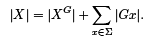In this case, the G-set is X = G. The set of ﬁxed points is the center: XG = Z (G). The nontrivial orbits Σ consist of conjugacy classes with more than one element. The order of such a conjugacy class is (G : C (x)), where C (x) is the centralizer of x. (I must take one such x for each nontrivial orbit.) Therefore,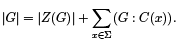This is called the class equation.

Example. Let G = S3 . The center of S3 is Z (S3) = {id}, and the nontrivial conjugacy classes are

{(1 2), (1 3), (2 3)}, {(1 2 3), (1 3 2)}.

Take one element from each nontrivial class: say (1 2) and (1 2 3). Their centralizers are

C ((1 2)) = {id, (1 2)} and C ((1 2 3)) = {id, (1 2 3), (1 3 2)}.

Thus,

|Z (S3)| + (S3 : C ((1 2))) + (S3 : C ((1 2 3))) = 1 + 3 + 2 = 6 = |S3|.

Example. Consider the group Q = {±1, ±i, ±j, ±k} of quaternions. Here are the conjugacy classes:

central elements: {1}, {−1}

nontrivial conjugacy classes: {i, −i}, {j, −j }, {k, −k}

I found these by direct computation. For example, the conjugates of i are: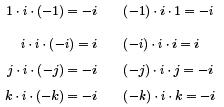Hence, {i, −i} is a complete conjugacy class.

In the class equation, the sum is taken over a set of representatives Σ for the nontrivial conjugacy classes. In this example, for instance, I could take Σ = {i, j, k} or Σ = {−i, −j, k} or . . . — i.e. any three elements, one from each of the (nontrivial) classes {i, −i}, {j, −j }, {k, −k}.

Finally, note that |Z (Q)| = 2, and the nontrivial classes have 2, 2, and 2 elements, respectively. The class equation says (correctly) that

2 + (2 + 2 + 2) = 8.

Before I prove the next result, I’ll review some things that are apparent from the derivation of the class equation. I had G acting on itself by conjugation. Then an orbit Gx (which does not mean “G times x” in this case!) is the conjugacy class of x — i.e. the set of elements conjugate to x. Assuming that G is ﬁnite, the order of the orbit equals the index of of the isotropy group, so |Gx| = (G : C (x)), where C (x) is the centralizer of x — the set of elements which commute with x.

In the Σx∈Σ (G : C (x)) term, I’m summing over conjugacy classes with more than one element. This implies two things:

(a) (G : C (x)) > 1, because (G : C (x)) equals the number of elements in the conjugacy class.

(b) |C (x)| < |G|, since |G| = (G : C (x))|C (x)|, and (G : C (x)) > 1.

Deﬁnition. Let p be prime. G is a p-group if for all g ∈ G, g ≠ 1, the order of g is pn for some n > 0. (n may be diﬀerent for diﬀerent elements.)

Finite p-groups are an important class of ﬁnite groups. Their structure is described in some detail by Sylow theory. Sylow theory is also an important tool in determining the structure of arbitrary ﬁnite groups. For example, it provides tools that helps answer questions like: How many diﬀerent groups of order 20 are there?

As applications of the class equation, I’ll look at some results that are “preliminaries” to Sylow theory. The ﬁrst result says that if p is prime and p | |G|, then G has an element of order p. Note that if n is not prime, n | |G| does not imply the existence of an element of order n. An easy counterexample: |Z3 × Z3 | = 9, which is divisible by 9, but Z3 × Z3 has no elements of order 9.

Lemma. Let G be a ﬁnite abelian group, and let p be a prime number which divides |G|. Then G has an element of order p.

Proof. Induct on |G|. Observe that Z2 has an element of order 2 and Z3 has an element of order 3 (and those are the only groups of order 2 and 3, respectively). This gets the induction started.
Now suppose n > 3, and assume the result is true for abelian groups of order less than n. Let |G| = n, p | n. I want to show that G has an element of order p.

Let x ∈ G, where x ≠ 0. If x has order pk for some k, then kx has order p. Therefore, assume that the order of x is not divisible by p.

Now (x) ≠ G, else x has order n, and n is divisible by p by assumption. Now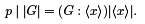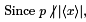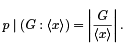Now |G/(x)| < |G| since |(x)| > 1, so G/(x) is an abelian group of order less than |G| whose order is divisible by p. By the induction hypothesis, G/(x) contains an element y + (x) of order p, where y ∈ G.
Suppose y has order m. Then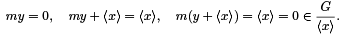Since m kills y + (x), it follows that m is divisible by the order p. Suppose m = kp. Then ky has order p.

This shows that G has an element of order p and completes the induction.

Theorem. (Cauchy) Let G be a ﬁnite group, and let p be a prime number which divides |G|. Then G has an element of order p.

Proof. Induct on |G|. Observe that Z2 has an element of order 2 and Z3 has an element of order 3 (and those are the only groups of order 2 and 3, respectively). This gets the induction started.

Now suppose n > 3, and assume the result is true for groups of order less than n. Let |G| = n, p | n. I want to show that G has an element of order p.

Consider x ∈ Σ, so the conjugacy class of x contains more than one element. By the remarks preceding the lemma, |C (x)| < |G|. By induction, if p | |C (x)|, then C (x) has an element of order p. Since C (x) < G, I’ll then have an element of order p in G.

Therefore, assume that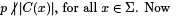Hence, p | (G : C (x)) for all x ∈ Σ. Look at the class equation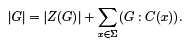p divides each term in the summation, and p divides |G| by assumption. Therefore, p | |Z (G)|. However, Z (G) is an abelian group, so the Lemma shows that it has an element of order p. Since Z (G) < G, G has an element of order p as well.

Corollary. Let p be prime. The order of a ﬁnite p-group is pn for some n > 0.

Proof. If G is a ﬁnite p-group, every element has order equal to a power of p. If |G| ≠ pn for some n, then q | |G|, where q is a prime number and q ≠ p. By Cauchy’s theorem, G has an element of order q, contradicting the fact that every element of a p-group has order equal to a power of p. Therefore, |G| = pn for some n.

Proposition. Let p be prime. The center of a nontrivial ﬁnite p-group is nontrivial.

Proof. Examine the class equation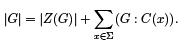Since |G| = pn for some n > 0, p | |G|. If x ∈ Σ, the remarks preceding the lemma above show that (G : C (x)) > 1. Since pn = |G| = (G : C (x))|C (x)|, it follows that p | (G : C (x)). Now p divides |G| and every term in the summation, so p divides |Z (G)|. Therefore, |Z (G)| ≠ 1.

Proposition. Let G be a group of order pn , where p is prime. Then G has a subgroup of order pk for 0 ≤ k ≤ n.

Proof. If n = 1, then G = Zp , which plainly has subgroups of orders p0 and p1.

Assume the result is true for groups of order pn−1, and suppose |G| = pn. The center Z (G) of G is nontrivial; since |Z (G)| | |G| = pn , it follows that |Z (G)| = pj for some j . By an earlier result, Z (G) contains an element x of order p.

Now x ∈ Z (G), so hxi ⊂ Z (G). Hence, (x) is normal. Form the quotient group G/(x). Now |G/(x)| = pn−1, so by induction G/(x) contains subgroups of order pk for 0 ≤ k ≤ n − 1. Each such subgroup has the form Hk /(x), where Hk is a subgroup of G containing (x).
Suppose then that |Hk /(x)| = pk . I have

|Hk | = |Hk /(x)||(x)| = pk · p = pk+1 .

Thus, H0 , H1 , . . . Hn−1 are subgroups of G orders p, p2 , . . . pn . Obviously, G contains a trivial subgroup of order p0 . This completes the induction step, and proves the result.

Offer running on EduRev: Apply code STAYHOME200 to get INR 200 off on our premium plan EduRev Infinity!

,

,

,

,

,

,

,

,

,

,

,

,

,

,

,

,

,

,

,

,

,

;